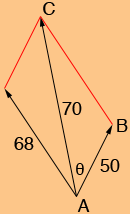SEARCH HOMEMath Central Quandaries & QueriesQuestion from Rita, a student: Before answering the question below, what exactly is, in basic words, resultant force? QUESTION: Two forces of 50 and 68 pounds act on a body to produce a resultant force of 70 pounds. Find, to the nearest 10 minutes or nearest tenth of a degree, the angle formed between the resultant force and the smaller force. Also, what does the phrase "to the nearest 10 minutes" actually mean?Hi Rita,

Suppose your friend Lisa is sitting on the ice in a skating rink and you and another friend Chelsea are going to push her so that she slides across the rink. You can't both get directly behind her so you push in slightly different directions. Thus you apply a force in one direction and Chelsea applies a force in a slightly different direction. Lisa will move in a direction between the direction you are pushing and the direction Chelsea is pushing. The force on Lisa which is the result of the two forces you and Chelsea apply is called the resultant. If you represent these forces by vectors then the resultant vector is the sum of the two vector forces applied by you and Chelsea.

In the problem you sent us you are given the magnitudes of these three vectors but not the directions. Here is my vector diagram.Thus you know the lengths of the three sides of the triangle ABC and hence you can find the measure of the angle θ using the Law of Cosines.

When you use the Law of Cosines and solve for cos(θ) you will need to use the inverse cosine function to find θ. If you are using a calculator to do this you will obtain a decimal number, like perhaps 14.76923 degrees. So to the nearest tenth of a degree that's 14.8 degrees. Angles are sometimes measured in degrees, minutes and seconds similar to the way we measure time in hours, minutes and seconds. There are 60 minutes in a degree and 60 seconds in a minute. Thus the angle I mentioned above, 14.76923 degrees is 14 degrees and 0.76923 × 60 = 46.1538 minutes. To the nearest 10 minutes that's 14 degrees 50 minutes.

I hope this helps,
HarleyMath Central is supported by the University of Regina and The Pacific Institute for the Mathematical Sciences.